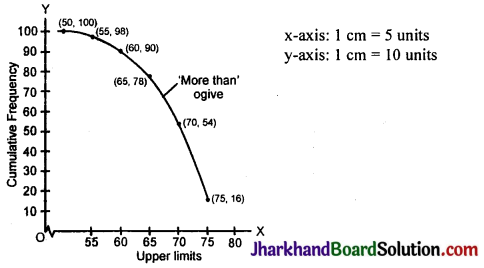# JAC Class 10 Maths Solutions Chapter 14 Statistics Ex 14.4

Jharkhand Board JAC Class 10 Maths Solutions Chapter 14 Statistics Ex 14.4 Textbook Exercise Questions and Answers.

## JAC Board Class 10 Maths Solutions Chapter 14 Statistics Exercise 14.4

Question 1.
The following distribution gives the daily income of 50 workers of a factory: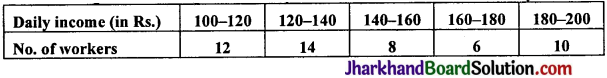Convert the distribution above to a less than type cumulative frequency distribution, and draw its ogive.
Solution: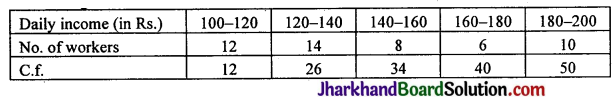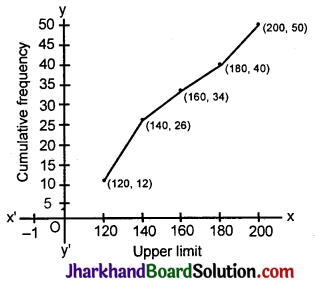Question 2.
During the medical check-up of 35 students of a class, their weights were recorded as follows:

 Weight (in kg) No. of students Less than 38 0 Less than 40 3 Less than 42 5 Less than 44 9 Less than 46 14 Less than 48 28 Less than 50 32 Less than 52 35

Draw a less than type ogive for the given data. Hence obtain the median weight from the graph and verify the result by using the formula.
Solution:

 Weight (in kg) Frequency Cumulative frequency 36 – 38 0 0 38 – 40 3 3 40 – 42 2 5 42 – 44 4 9 44 – 46 5 14 = cf 46 – 48 14 = f 28 48 – 50 4 32 50 – 52 3 35 n = 35

$$\frac{\mathrm{n}}{2}=\frac{35}{2}=17.5$$
Plot the points (38, 0) (40, 3) (42, 5) (44, 9) (46, 14) (48, 28) (50, 32) (52, 35)
Median = l + $$\left[\frac{\frac{\mathrm{n}}{2}-\mathrm{cf}}{\mathrm{f}}\right]$$ × h
= 46 + $$\left[\frac{17.5-14}{14}\right]$$ × 2
= 46 + $$\frac{3.5 \times 2}{14}$$
= 46 + 0.5= 46.5.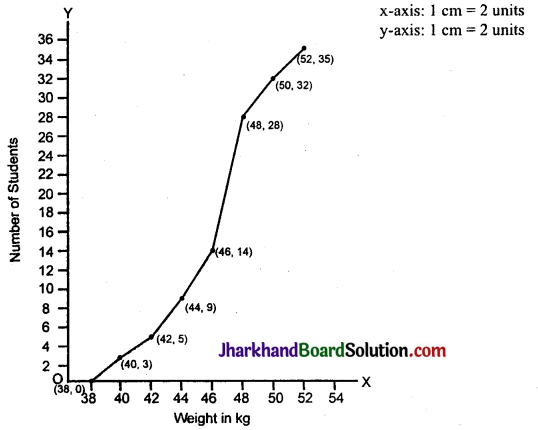Question 3.
The following table gives production yield per hectare of wheat of 100 farms of a village: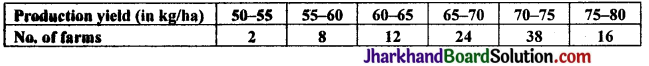Change the distribution to a more than type distribution, and draw its ogive.
Solution:

 Production yield (in kg/hec) Number of farms c.f. More than 50 2 100 More than 55 8 98 More than 60 12 90 More than 65 24 78 More than 70 38 54 More than 75 16 16

∴ Co-ordinate points are (50, 100), (55, 98), (60, 90), (65, 78), (70, 54) and (75, 16).